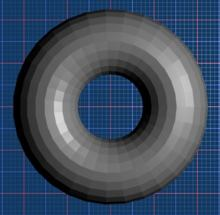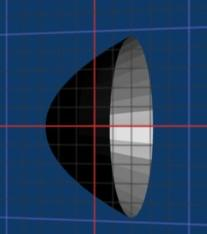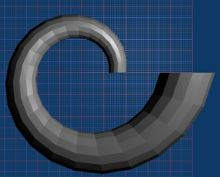# Maths - Parameterisation

We can often specify a surface in terms of a set of coordinates (often orthogonal coordinates but cylindrical and spherical coordinates can also be useful). So, for example, a surface of a sphere can be specified by:

r²=x²+y²+z²

We could use this to plot the surface, we might choose to step through two of the dimensions and calculate the third parameter to give a point on the surface of the sphere using:

z = √(r²-x²-y²)

there are possible problems with this:

• Not all values of x,y will produce a point on the sphere.
• Other values of x,y will produce two points corresponding to the front and back faces of the surface.
• Different parts of the surface will be covered in different levels of detail, so the poles will have a much lower density of points than the equator.

In order to minimise these problems it often helps to express the surface in terms of a set of parameters other than the base coordinates:

Shape Parameters Limits

Sphere

r²=x²+y²+z²in spherical coordinates:

c1 = R
c2 = π*u
c3 = 2*π*v

convert to rectangular coordinates

x = c1 * math.sin(c2) * math.cos(c3)
y = c1 * math.sin(c2) * math.sin(c3)
z = c1 * math.cos(c2)

where:

0< u <1
0< v <1
Cylinder

Torusin cylindrical coordinates:

c1 =r0 + r1*cos(2*π*u)
c2 =2*π*v
z =r1*sin(2*π*u)

convert to rectangular coordinates

x = c1 * math.cos(c2)
y = c1 * math.sin(c2)

where:

0< u <1
0< v <1

Möbius stripx(u,v) = (1 + (v/2)*cos(u/2))*cos(u)
y(u,v) = (1 + (v/2)*cos(u/2))*sin(u)
z(u,v) = (v/2)*sin(u/2)
0<u<= 2*π
-1 < v < 1
Klein bottle (represented in 3 dimensions)

x(u,v) = sin(v)*(4 + 2*cos(u)*cos(t*v)- sin(2*u)*sin(t*v)
y(u,v) = cos(v)*(4 + 2*cos(u)*cos(t*v)- sin(2*u)*sin(t*v)
z(u,v) = 2*cos(u)*sin(t*v)+sin(2*u)*cos(t*v)

t = twist = 0.5

Note1: this is a 'figure-8' Klien bottle. The usual form is built from 4 parts.
Yet another version (Lawson-Klein) can be built from a helicoidal surface.

Note2: This can only be physically realised in four dimensions, since it must pass through itself without making a hole.

Circular helix

x=a*sin(t),
y=a*cos(t),
z=at/(2*π*c)

Lissajous curve

x= r*sin(theta*t)*cos(phi*t),
y= r*sin(theta*t)*sin(phi*t),
z = cos(phi*t)

A coil winding around a torusin cylindrical coordinates:

c1=R0 + R1*E0*cos(2*π*n1*v+phi1)+R2*E1*cos(2*π*n2*u+2*π*n1*v+phi1)
c2=2*π*v
z=R1*E0*sin(2*π*n1*v+phi1)+R2*E1*sin(2*pi*n2*u+2*pi*n1*v+phi1)

convert to rectangular coordinates

x = c1 * math.cos(c2)
y = c1 * math.sin(c2)

where:

R1=0.3 = Radius of the section of the torus
R2=0.075 = Radius of the coil
n1=3.3 = Number of full circles of the coil around the torus
phi1 = 0 = Starting angle of the coil
n2= 1= Number of points for the coil section
alpha0=1=Exponential factor for the torus section R1
alpha1=1=Exponential factor for the coil radius R2

0< u <1

-2< v <1

coil sphere 1

in spherical coordinates:

c1=r0+r1t*cos(2*π*u)
c2=(r1t/r0)*sin(2*π*u)+π*v/(2*n)+π/2
c3=2*π*v

convert to rectangular coordinates

x = c1 * math.sin(c2) * math.cos(c3)
y = c1 * math.sin(c2) * math.sin(c3)
z = c1 * math.cos(c2)

where:

n=3=Coils
r1t=r1*((abs(v)-n)/n)²

0< u <1
-n< v <n

coil sphere 2

in spherical coordinates:

c1=r0+r1*tap*cos(2*π*nth*v+thphi)+r2*tap*cos(2*π*u)
c2=r1*tap*sin(2*π*nth*v+thphi)+r2*tap*sin(2*π*u)+π*v/(2*n)+π/2
c3=2*π*v

convert to rectangular coordinates

x = c1 * math.sin(c2) * math.cos(c3)
y = c1 * math.sin(c2) * math.sin(c3)
z = c1 * math.cos(c2)

where:

n=3=Coils
tap=((abs(v)-n)/n)²

0< u <1
-n< v <n

A Paraboloid (Revolution type)

z = a(x²+y²)in cylindrical coordinates:

c1 = u
c2 = 2*π*v
z = a*u²

convert to rectangular coordinates

x = c1 * math.cos(c2)
y = c1 * math.sin(c2)

where:

a=1

0< u <1

0< v <1

A Paraboloid

z = a(x²+y²)

x = u
y = v
z = a*(u²-v²)

where:

a=1

-1< u <1

-1< v <1

Shellin cylindrical coordinates:

c1 = (r0 + Sp*cos(2*π*u))*E0
c2 = 2*π*v
z = d*E0+Sp*sin(2*π*u)*E0

convert to rectangular coordinates

x = c1 * math.cos(c2)
y = c1 * math.sin(c2)

where:

r0=0.22=Radius of the main winding up coil
r1=0.20=Radius of the circle defining the section
r2=0.5=Spike length
alpha=0.30=Exponential ratio of the spiral
d=-1.10=Vertical displacement of each spiral turn
N0=6=Number of spikes across
M0=8=Sharpness of the spikes across
N1=10.5347=Number of the spikes along (each turn)
M1=12=Sharpness of the spikes across
beta=1.5=Winding of the spikes
a=10=Faloff of spikes
Sp=(r1+r2*Gauss*SpAlong*SpAcross)=Spikes
E0=exp(alpha*v)=Exponential factor
Gauss=exp(-a*(cos(2*π*u)-1.0)²)=Gaussian Spike tapering
SpAlong=((sin(N1*2*π*v)+1)/2.0)M1=Spikes Along formula
SpAcross=((sin(N0*2*π*u+beta*2*π*v)+1)/2.0)M0=Spikes across Formula

0< u <1

-9< v <1

Logarithmic Spiralin cylindrical coordinates:

c1 =r0*E+r1*E*cos(2*π*u)
c2 =2*π*v
z =d*E+r1*E*sin(2*π*u)

convert to rectangular coordinates

x = c1 * math.cos(c2)
y = c1 * math.sin(c2)

where:

alpha0=0.15=Exponential factor
d=0.75=Step along z
E=exp(alpha0*2*π*v)=Exponential behavior

0< u <1
0< v <1

 metadata block see also: normals Correspondence about this page Book Shop - Further reading. Where I can, I have put links to Amazon for books that are relevant to the subject, click on the appropriate country flag to get more details of the book or to buy it from them.3D Math Primer - Aimed at complete beginners to vector and matrix algebra. Other Math Books